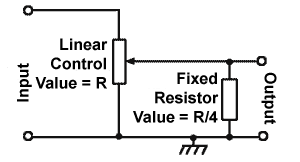# Logarithmic and Linear Controls

• After studying this section, you should be able to:
• • Describe the uses for linear & logarithmic (taper) track potentiometers.
• • Describe the operation of linear & logarithmic track potentiometers.
• • Describe the conversion of linear to logarithmic tracks.

## Two Types of Track

Two types of potentiometers with different tracks are available. These are Linear (Lin) or Logarithmic (Log) tracks.

With linear potentiometers, the resistance between one end of the track and the wiper varies at a constant rate as the slider is moved along the track. In logarithmic types, the change in resistance is much less at one end of the track to the other. Fig. 2.6.1 shows a graph of the change in resistance for given equal movements of the slider follows an (approximate) logarithmic curve; this effect is often referred to as ‘taper’.## Logarithmic (Taper) Potentiometers

Logarithmic potentiometers are used as volume controls in audio equipment because the response of the human ear to the loudness of sound is also logarithmic. Using a log pot therefore gives the effect that a setting of full volume on the control sounds twice as loud as a setting of half volume. A linear pot used as a volume control would give large apparent changes in loudness at low volume settings, with little apparent change over the rest of the control´s range.

The action of a logarithmic potentiometer as shown above is only approximately logarithmic and in fact in many less expensive commercial pots the logarithmic track is actually made up of two sections of linear track, each having a different resistance. This creates an output which rises slowly at first due to a high resistance track, then about half way along, the track changes to low resistance, giving a fast rising output from the slider. Not really logarithmic, but a close enough approximation to fool the ears.

## Lin to Log Conversion### Fig. 2.6.2 Converting a Linear Control to Logarithmic

It is also possible to get a close approximation to logarithmic operation by modifying the potentiometer as shown in Fig. 2.6.2. A fixed resistor having a value about a quarter of the potentiometer value is connected from the low (ground) end of the potentiometer to the slider. As the control is adjusted this resistor bypasses the potentiometer track by varying amounts giving a curved response similar to true log operation.